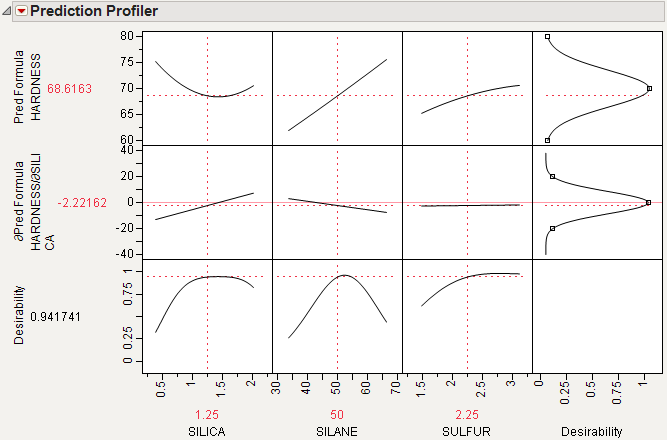Publication date: 08/13/2020

## Noise Factors

Note: Noise factor optimization is also available in the Prediction Profiler, Contour Profiler, Custom Profiler, and Mixture Profiler.

Noise factors (robust process engineering) enables you to produce acceptable products reliably, despite variation in the process variables. Even when your experiment has controllable factors, there is a certain amount of uncontrollable variation in the factors that affects the response. This is called transmitted variation. Factors with this variation are called noise factors. Some factors you cannot control at all, like environmental noise factors. The mean for some factors can be controlled, but not their standard deviation. This is often the case for intermediate factors that are output from a different process or manufacturing step.

A good approach to making the process robust is to match the target at the flattest place of the noise response surface. Then, the noise has little influence on the process. Mathematically, this is the value where the first derivatives of each response with respect to each noise factor are zero. JMP computes the derivatives for you.

Figure 2.11 Noise Factor ExampleTo analyze a model with noise factors:

1. Fit the appropriate model (for example, using the Fit Model platform).

2. Save the model to the data table with the Save > Prediction Formula command.

3. Launch the Profiler (from the Graph menu).

4. Assign the prediction formula to the Y, Prediction Formula role and the noise factors to the Noise Factor role.

5. Click OK.

The resulting profiler shows response functions and their appropriate derivatives with respect to the noise factors. The derivatives are set to have maximum desirability at zero.

6. Select Optimization and Desirability > Maximize Desirability from the Profiler menu.

This finds the best settings of the factors, balanced with respect to minimizing transmitted variation from the noise factors.